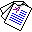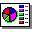# Equivalence in Abductive Logic

## Katsumi Inoue and Chiaki Sakama

Proceedings of the 19th International Joint Conference on Artificial Intelligence (IJCAI-05), pages 472-477, Morgan Kaufmann Publishers, 2005.

## Abstract

We consider the problem of identifying equivalence of two knowledge bases which are capable of abductive reasoning. Here, a knowledge base is written in either first-order logic or nonmonotonic logic programming. In this work, we will give two definitions of abductive equivalence. The first one, explainable equivalence, requires that two abductive programs have the same explainability for any observation. Another one, explanatory equivalence, guarantees that any observation has exactly the same explanations in each abductive framework. Explanatory equivalence is a stronger notion than explainable equivalence. In first-order abduction, explainable equivalence can be verified by the notion of extensional equivalence in default theories. In nonmonotonic logic programs, explanatory equivalence can be checked by means of the notion of relative strong equivalence. We also show the complexity results for abductive equivalence.Full Paper (pdf 94K)Slide (pdf 143K)